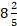• Save

# Time and Work Problems with Solutions for Bank ExamsUsually, students are puzzled in time and work problems of the competitive exams because they don't know the proper way to solve the time and work questions or equations. These time and work questions frequently asked in ssc and bank exams.

So here in this blog, you can easily learn how to solve the time and work problem with solutions and different-2 examples. You can practice also time and work questions with answers after learning solutions of time and work. Keep practice and improve your confidence as well.

## Time and Work Problems with Solutions for Competitive Exams:

Q.1. Worker A takes 8 hours to do a job. Worker B takes 10 hours to do the same job. How long should it take both A and B, working together but independently, to do the same job?

Solution:

$$A's \ same \ 1 \ hours's \ work = {1\over8}$$

$$B's \ same \ 1 \ hours's \ work = {1\over10}$$

$$(A+B)'s \ same \ 1 \ hours's \ work = {1\over8}+{1\over10}={9\over40}$$

$$∴ Both \ A \ and \ B \ will \ finish \ the \ work \ in {40\over9}=4{4\over9}days$$

Q.2. A and B together can complete a piece of work in 4 days. If A alone can complete the same work in 12 days, in how many days can B alone complete that work?

Solution:

$$(A+B)'s \ 1 \ days \ work = {1\over4}$$

$$A's \ 1 \ days \ work = {1\over12}$$

$$∴B's \ 1 \ days \ work = {1\over4}-{1\over12} ={1\over6}$$

Hence, B alone can complete work in 6 days.

Q.3. A can do a piece of work in 7 days of 9 hours each and B can do it in 6 days of 7 hours each. How long will they take to do it, working togetherhours a day ?

Solution:

A can complete the work in (7×9)=63 hours.

B can complete the work in (6×7)=42 hours.

∴ A’s 1 hour’s $$Work \ = {1\over63}$$

and B’s 1 hour’s $$Work \ = {1\over42}$$.

(A+B)’s 1 hours   $$Work= \left({1\over63}+{1\over42}\right)={5\over126}$$

∴ Both will finish the $$Work \ in = \left({126\over5}\right)hrs$$

Number of days ofhrs   $$each=\left({126\over5}×{5\over42}\right)=3 days$$

Q.4. A does a work in 10 days and B does the same work in 15 days. In how many days they together will do the same work?

Solution:

A’s 1 day’s $$Work= {1\over10}$$

And B’s day’s $$Work= {1\over15}$$

∴ (A+B)’s 1 day’s   $$Work= \left({1\over10}+{1\over15}\right)={1\over6}$$

So, both together will finish the work in 6 days.

Q.5. A can finish a work in 18 days and B can do the same work in half the time taken by A. Then, working together, what part of the same work they can finish in a day?

Solution:

A’s 1 day’s $$Work= {1\over18}$$

And B’s day’s $$Work= {1\over9}$$

∴  (A+B)’s 1 day’s    $$Work= \left({1\over18}+{1\over9}\right)={1\over6}$$

Ask me in the comment section, if you face any problem while solving important Time and Work questions. Visit next page for more Time and Work problems.